NEET  >  DC Pandey Solutions: Semiconductor

# DC Pandey Solutions: Semiconductor - Notes | Study Physics Class 12 - NEET

 1 Crore+ students have signed up on EduRev. Have you?

Introductory Exercise 32.1

Ques 1: Carbon, silicon and germanium have four valence electrons each. These are characterised by valence and conduction bands separated by energy band-gap respectively equal to (Eg)C, (Eg)Si and (Eg)Ge. Which of the following statements is true?
(a) (Eg)Si < (Eg)Ge < (Eg)C
(b) (Eg)< (Eg)Ge > (Eg)Si
(c) (Eg)> (Eg)Si > (Eg)Ge
(d) (Eg)= (Eg)S= (Eg)Ge
Ans:
(c)

Introductory Exercise 32.2

Ques 1: In an unbiased p - n junction, holes diffuse from the p -region to n-region because
(a) free electrons in the n-region attract them
(b) they move across the junction by the potential difference
(c) hole concentration in p -region is more as compared to n-region
(d) All of the above
Sol:
(c)

Ques 2: When a forward bias is applied to a p-n junction. lt
(a) raises the potential barrier
(b) reduces the majority carrier current to zero
(c) lowers the potential barrier
(d) All o f the above
Sol: (c)

Introductory Exercise 32.3

Ques 1: For transistor action, which of the following statements are correct?
(a) Base, emitter and collector regions should have similar size and doping concentrations
(b) The base region must be very thin and lightly doped
(c) The emitter junction is forward biased and collector junction is reverse biased
(d) Both the emitter junction as well as the collector junction are forward biased
Ans:
(b,c)

Ques 2: For a transistor amplifier, the voltage gain
(a) remains constant for all frequencies
(b) is high at high and low frequencies and constant in the middle frequency range
(c) is low at high and low frequencies and constant at mid frequencies
(d) None of the above
Ans:
(c)

Ques 3: For a CE-transistor amplifier, the audio signal voltage across the collector resistance of 2 kΩ is 2 V. Suppose the current amplification factor of the transistor is 100. Find the input signal voltage and base current, if the base resistance is 1 kΩ.
Ans:
Vi = 0.01 V, ib = 10 μA

Introductory Exercise 32.4

Ques 1: Make the output waveform Y of the NAND gate for the following inputs A and B.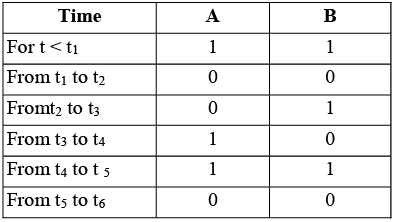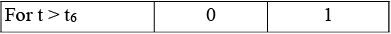Ans: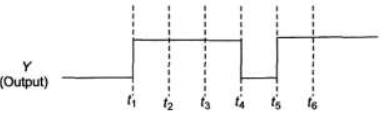Ques 2: You are given two circuits. Identify the logic operation carried out by the two circuits.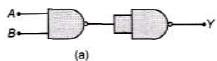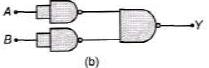Ans:
(a) AND (b) OR

The document DC Pandey Solutions: Semiconductor - Notes | Study Physics Class 12 - NEET is a part of the NEET Course Physics Class 12.
All you need of NEET at this link: NEET

## Physics Class 12

157 videos|452 docs|213 tests
 Use Code STAYHOME200 and get INR 200 additional OFF

## Physics Class 12

157 videos|452 docs|213 tests

### How to Prepare for NEET

Read our guide to prepare for NEET which is created by Toppers & the best Teachers

Track your progress, build streaks, highlight & save important lessons and more!

,

,

,

,

,

,

,

,

,

,

,

,

,

,

,

,

,

,

,

,

,

;